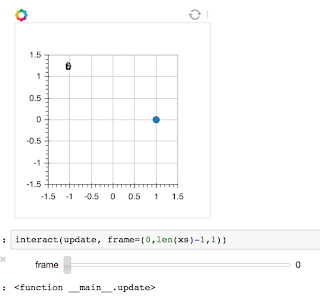# Updating textGlpyh.data_source.data via widget creates bad formatting

Demonstrated in the attached file. Any solutions? Workarounds?

I’ve been meaning to learn how to make similar interactive figures using the javascript method. Any chance that would be handled differently?

Thanks,

• gD

InteractiveFigure.ipynb (26.3 KB)Enter code here…

``

import numpy as np

from bokeh.io import push_notebook

import bokeh.plotting as bkP

import bokeh.models as bkM

from ipywidgets import interact

import numpy as np

bkP.output_notebook()

**** New cell ****

xs = np.cos(np.linspace(0, 2*np.pi, 2000))

ys = np.sin(np.linspace(0, 2*np.pi, 2000))

frame = 0

p = bkP.figure(plot_width=300,plot_height=300,tools=‘reset’)

p.x_range = bkM.Range1d(-1.5,1.5)

p.y_range = bkM.Range1d(-1.5,1.5)

frame = 0

theCircle = p.circle([xs[frame]],

[ys[frame]],

name = ‘circle’,size=10)

frString = ‘fr=%1u’ % frame

tBox = p.text(text = [frString],x = -1.1, y = 1.1)

bkP.show§

def update(frame=0):

theCircle.data_source.data[‘x’] = [xs[frame]]

theCircle.data_source.data[‘y’] = [ys[frame]]

frString = ‘fr=%1u’ % frame

tBox.data_source.data = {‘text’: frString}

push_notebook()

**** New cell ****

interact(update, frame=(0,len(xs)-1,1))

Let me increase my chances of a response by pasting the code and a screenshot. Notice that the characters in the top left of the figure are all on top of one another. Negative kerning!

thanks,

• gD
···

On Thursday, January 28, 2016 at 11:30:13 AM UTC-5, Gabriel Diaz wrote:

Demonstrated in the attached file. Any solutions? Workarounds?

I’ve been meaning to learn how to make similar interactive figures using the javascript method. Any chance that would be handled differently?

Thanks,

• gD